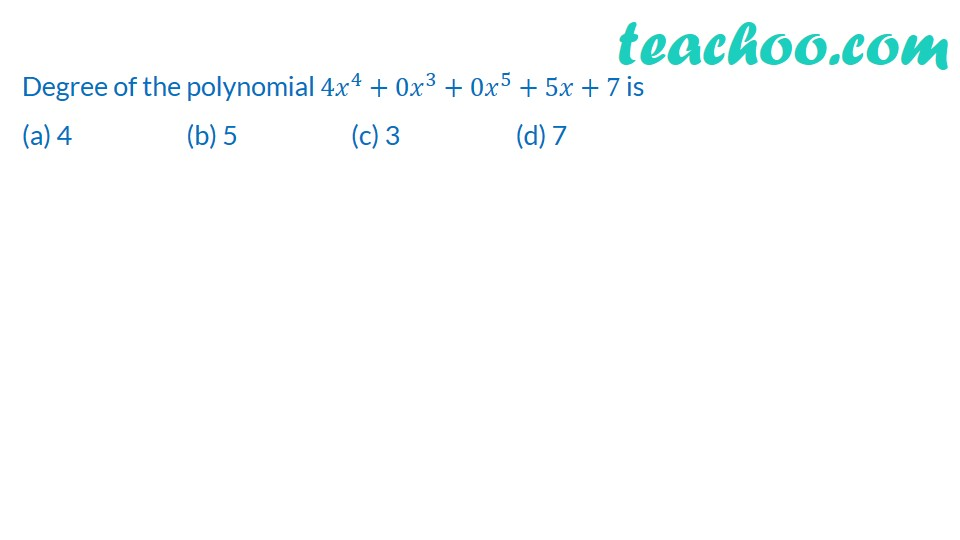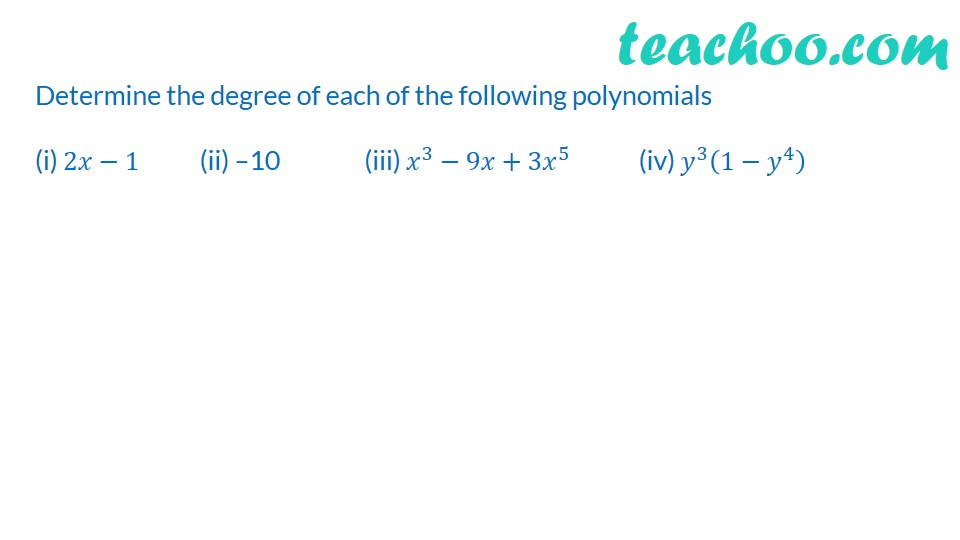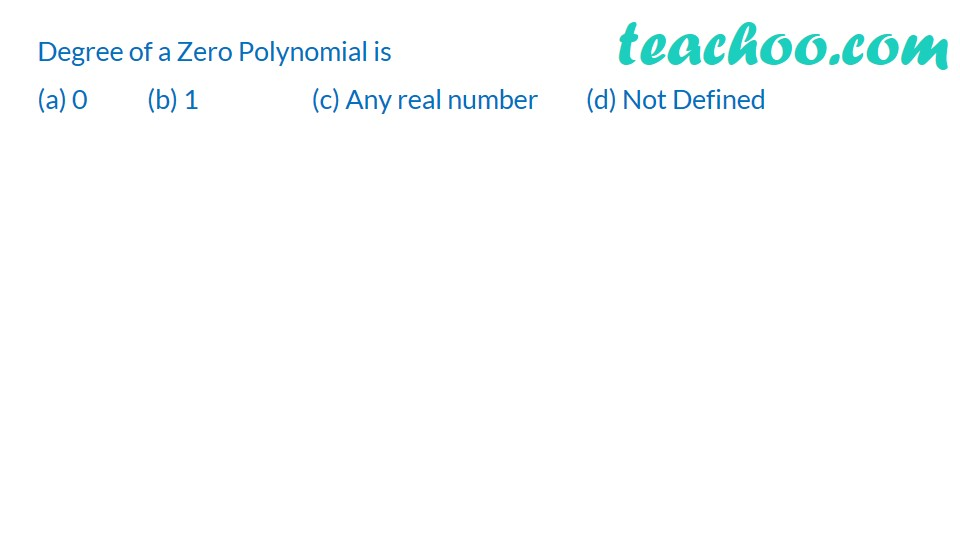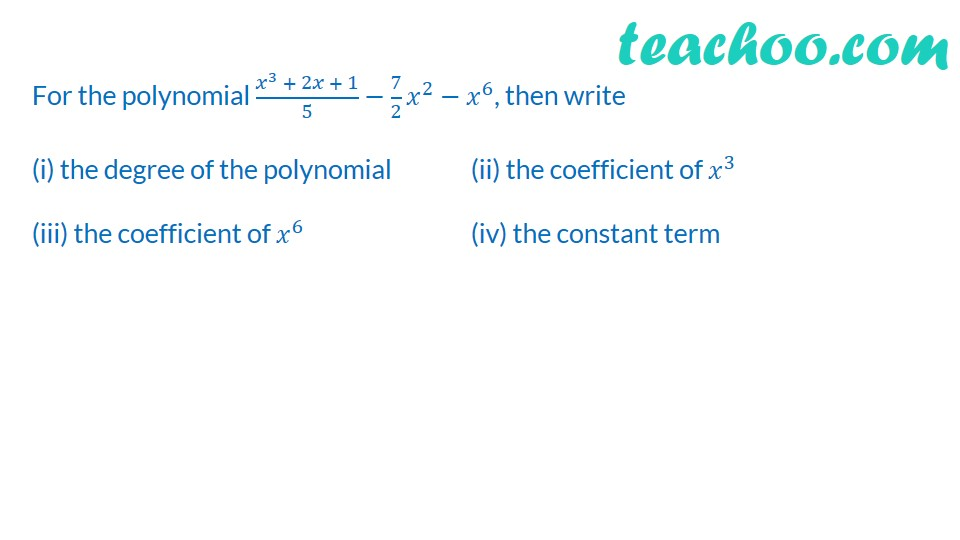Degree & Coefficient of a polynomial

Chapter 2 Class 9 Polynomials
Concept wiseLearn in your speed, with individual attention - Teachoo Maths 1-on-1 Class

### Transcript

√2 is a polynomial of degree (a) 2 (b) 0 (c) 1 (d) 1/2Degree of the polynomial 〖4𝑥〗^4+0𝑥^3+0𝑥^5+5𝑥+7 is (a) 4 (b) 5 (c) 3 (d) 7Determine the degree of each of the following polynomials (i) 2𝑥−1 (ii) –10 (iii) 𝑥^3−9𝑥+3𝑥^5 (iv) 𝑦^3 (1−𝑦^4 )Degree of a Zero Polynomial is (a) 0 (b) 1 (c) Any real number (d) Not Defined Give an example of (i) A monomial of degree 5. (ii) A binomial of degree 8. (iii) A trinomial of degree 4. (iv) A monomial of degree 0. For the polynomial (𝑥^3 + 2𝑥 + 1)/5−7/2 𝑥^2−𝑥^6, then write (i) the degree of the polynomial (ii) the coefficient of 𝑥^3 (iii) the coefficient of 𝑥^6 (iv) the constant term